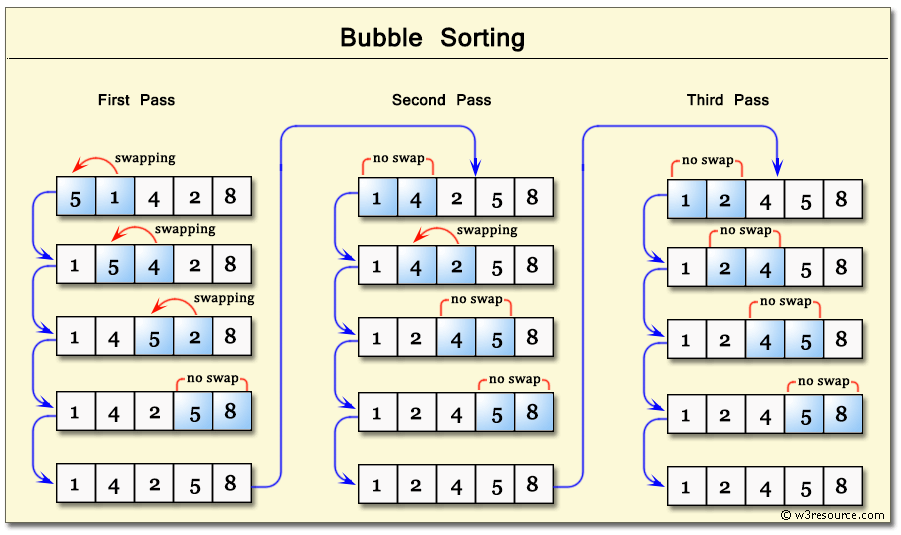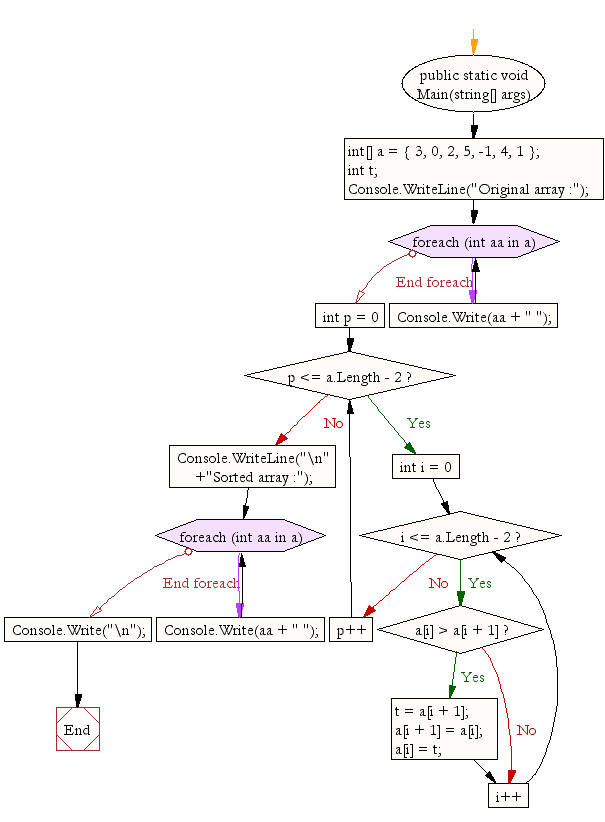﻿ C# - Bubble sort

# C#: Bubble sort

## C# Sharp Searching and Sorting Algorithm: Exercise-3 with Solution

Write a C# Sharp program to sort a list of elements using Bubble sort.

According to Wikipedia "Bubble sort, sometimes referred to as sinking sort, is a simple sorting algorithm that repeatedly steps through the list to be sorted, compares each pair of adjacent items and swaps them if they are in the wrong order. The pass through the list is repeated until no swaps are needed, which indicates that the list is sorted. The algorithm, which is a comparison sort, is named for the way smaller elements "bubble" to the top of the list. Although the algorithm is simple, it is too slow and impractical for most problems even when compared to insertion sort. It can be practical if the input is usually in sort order but may occasionally have some out-of-order elements nearly in position."

Step by step pictorial presentation :Sample Solution:-

C# Sharp Code:

``````using System;
public class Bubble_Sort
{
public static void Main(string[] args)
{
int[] a = { 3, 0, 2, 5, -1, 4, 1 };
int t;
Console.WriteLine("Original array :");
foreach (int aa in a)
Console.Write(aa + " ");
for (int p = 0; p <= a.Length - 2; p++)
{
for (int i = 0; i <= a.Length - 2; i++)
{
if (a[i] > a[i + 1])
{
t = a[i + 1];
a[i + 1] = a[i];
a[i] = t;
}
}
}
Console.WriteLine("\n"+"Sorted array :");
foreach (int aa in a)
Console.Write(aa + " ");
Console.Write("\n");

}
}
```
```

Sample Output:

```Original array :
3 0 2 5 -1 4 1
Sorted array :
-1 0 1 2 3 4 5
```

Flowchart:C# Sharp Code Editor: